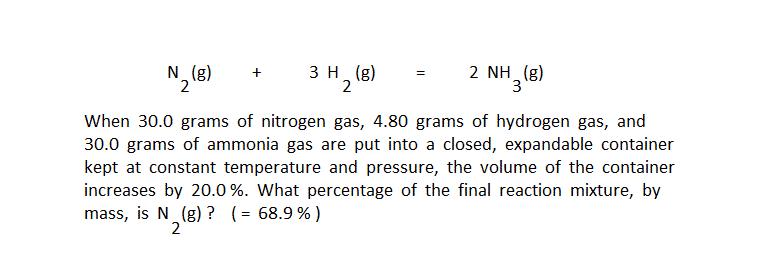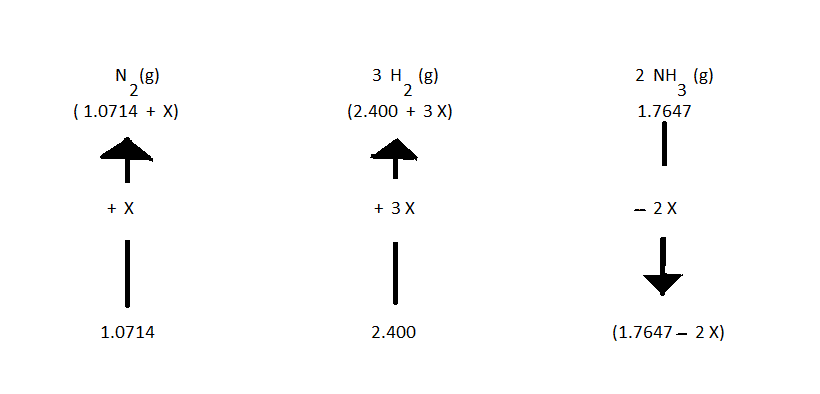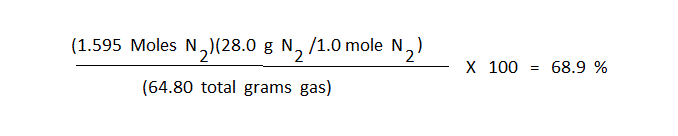This is a booger problem because the given volume change has to be used logically to solve the problem. It is a "spaghetti sauce" problem because all products and all reactants are simultaneously together in a reaction mixture that cannot be at chemical equilibrium (it's "all in there!" just like the spaghetti sauce). The first thing needed is to determine the direction in which the reaction proceeds to establish equilibrium.The volume of the gas depends on temparature, pressure, and moles of gas. But T and P are both held constant, so to increase the volume, the reaction must increase the moles of gas in the container. The reaction must proceed in the reverse direction to increase moles of gas. The preliminary arrow diagram can now be set up, with arrows being drawn to show that the moles of product in the reaction mixture is decreasing, and moles of reactants are increasing.The volume of the gas increases by 20.0 % because the total moles of gas in the container increases by 20.0 %. With the reaction proceeding in the reverse direction, net change in moles of gas is 2Xact:2X=(1.0714 + 2.400 + 1.7647 total moles gas)

Solving this equation,X=0.5236

The final required answer is calculated by computing the final moles of N2, converting that value to grams N2, then giving the percentage obtained by dividing grams of N2(g) by 64.80 total grams of gas.The "special feature" of the spaghetti sauce problem is that all substances in the reaction are present when the reaction is not at equilibrium, so some information in the problem must be used logically to determine the direction in which the reaction proceeds to establish equilibrium. The same information is usually used to calculate the value of Xact for the arrow diagram.